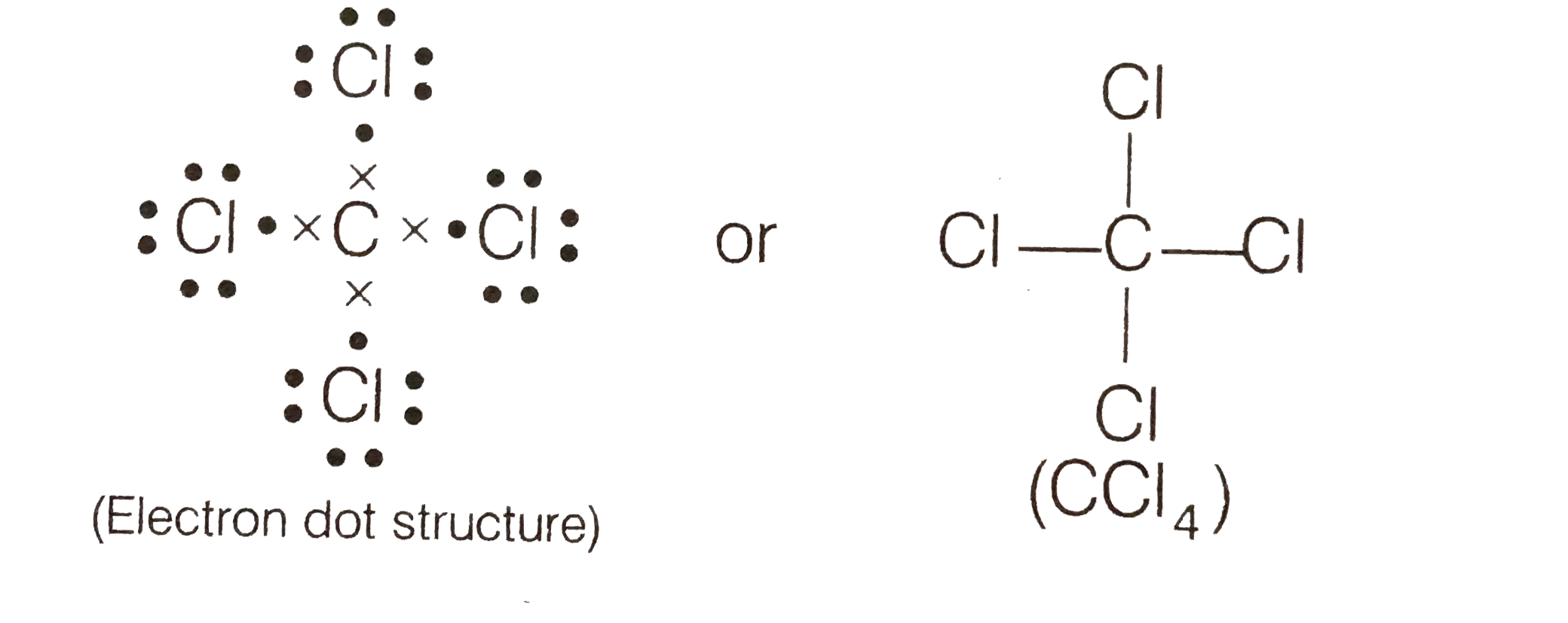# (a) Write the formula and draw electron dot structure of carbon tetrachloride. (b) What is saponification ? Write the reaction involved in this proces

412 views

closed
(a) Write the formula and draw electron dot structure of carbon tetrachloride.
(b) What is saponification ? Write the reaction involved in this process.

by (72.0k points)
selected

(a) The formula of carbon tetrachloride is C Cl_(4). Its electron dot structure is as follows(b) Saponification Alkaline hydrolysis of an ester to give the salt corresponding acid and the alcohol is called saponification. It is reverse of esterification reaction.
e.g.,
underset("Ethyl ethanoate")(CH_(3)-overset(O)overset(||)(C)-OCH_(2)CH_(3))+underset("Sodium hydroxide")(NaOH)underset(("asponification"))overset("Heat")tounderset("Sodium ethanoate")(CH_(3)-overset(O)overset(||)C-ONa)+underset("Ethanol")(CH_(3)CH_(2))-OH
Dehydration means removal of a molecule of water. When ethanol is heated with conc.
H_(2)SO_(4) at 443 K, it undergoes dehydration to form ethene.
underset("Ethanol")(CH_(3)CH_(2)OH)underset(("dehydration"))overset("Conc."H_(2)SO_(4),443K)tounderset("Ethene")(CH_(3)=CH_(2))+H_(2)O